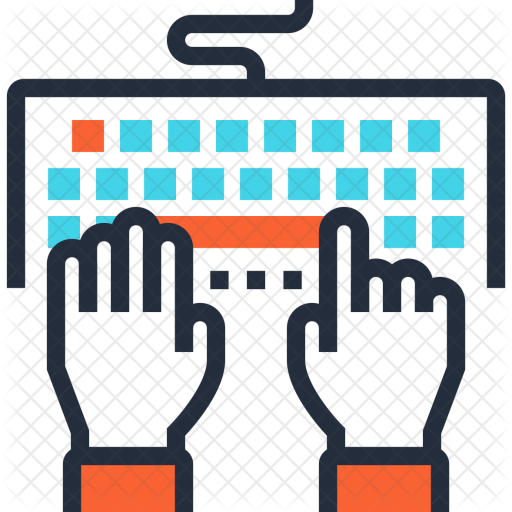# Writing R Functions

In this lesson, you will learn to:

• Write your own functions in R

• Make good decisions about function arguments and returns

• Include side effects and/or error messages in your functions

• Use good R code styleTime Estimates:Videos: 30 minReadings: 10-30 minActivities: 60-90 minCheck-ins: 4

## Writing FunctionsRequired Video: Writing FunctionsRequired Tutorial: Primer: Writing FunctionsRecommended Reading: R4DS: FunctionsCheck-In 1: Simple Functions

Write a function called `times_seven()` which takes a single argument and multiplies by 7.

This function should check that the argument is numeric.

This function should also excitedly announce (print) “I love sevens!” if the argument to the function is a 7.Check-In 2: Predicting Function Behavior

``````add_or_subtract <- function(first_num, second_num = 2, type = "add") {

first_num + second_num
} else if (type == "subtract") {
first_num - second_num
} else {
}

}``````

Question 1: What will be returned by each of the following?

1. 1
2. -1
3. 30
4. An error defined in the function `add_or_subtract`
5. An error defined in a different function, that is called from inside `add_or_subtract`
``````add_or_subtract(5, 6, type = "subtract")

Question 2:

Consider the following code:

``````first_num <- 5
second_num <- 3

result <- 8

result <- add_or_subtract(first_num, second_num = 4)

1. `first_num`
2. `second_num`
3. `result`
4. `result_2`